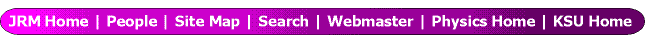Charge transfer in slow collisions of O^8+ and Ar^8+ ions with H(1s) below 2 keV/amu

Teck-Ghee Lee
(Physics Div. ORNL, Oak Ridge, TN 37831),

M. Hesse
(Physique Quantique CP165/82, Universite Libre de Bruxelles,),

Anh-Thu Le, C.D. Lin
(epartment of Physics, Kansas State University, Manhattan, Kansas 66506)

We calculated the charge transfer cross sections for O^8++H collisions for energies from 1 eV/amu to 2 keV/amu using the recently developed hyperspherical close-coupling method. In particular, the discrepancy for electron capture to the n = 6 states of O^7+ from the previous theoretical calculations is further analyzed. Our results indicate that at low energies (below 100 eV/amu) electron capture to the n = 6 manifold of O^7+ becomes dominant. The present results are used to resolve the long standing discrepancies from the different elaborate semiclassical calculations near 100 eV/amu. We have also carried out calculations for Ar^8++H collisions in the same energy range to analyze the effect of the ionic core on the subshell cross sections. By using diabatic molecular basis functions, we show that converged results can be obtained with small numbers of channels.

This work was supported by the Chemical Sciences, Geosciences and Biosciences Division,
Office of Basic Energy Sciences, Office of Science, U.S. Department of Energy.

Submitted to DAMOP, May 2004 in Tucson, AZ.